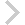FICO Xpress Optimization Examples Repository
 FICO Optimization Community FICO Xpress Optimization HomeSludge production planning solved by recursion

Description
The two model versions show how to solve a production planning problem via iterative LP solves (sludge.mos) or by using a nonlinear formulation (sludge2.mos).

Further explanation of this example: This model is discussed in Section 11.2.1 of the book 'J. Kallrath: Business Optimization Using Mathematical Programming - An Introduction with Case Studies and Solutions in Various Algebraic Modeling Languages' (2nd edition, Springer, Cham, 2021, DOI 10.1007/978-3-030-73237-0).

Source Files
By clicking on a file name, a preview is opened at the bottom of this page.

sludge.mos

(!*********************************************************************
Mosel Example Problems
======================

file sludge.mos

Sludge problem illustrating recursion

Example discussed in section 11.2.1 of
J. Kallrath: Business Optimization Using Mathematical Programming -
An Introduction with Case Studies and Solutions in Various Algebraic
Modeling Languages. 2nd edition, Springer Nature, Cham, 2021

author: S. Heipcke, June 2018

(c) Copyright 2020 Fair Isaac Corporation

you may not use this file except in compliance with the License.
You may obtain a copy of the License at

Unless required by applicable law or agreed to in writing, software
WITHOUT WARRANTIES OR CONDITIONS OF ANY KIND, either express or implied.
See the License for the specific language governing permissions and

*********************************************************************!)

model 'sludge'
uses "mmxprs"

declarations
I= 2                        ! Number of sources
J= 2                        ! Number of processing areas
K= 3                        ! Number of destinations (end points)
C= 2                        ! Number of components
RI=1..I
RJ=1..J
RK=1..K
RC=1..C

LAM: array(RC,RJ) of real   ! Assumed fraction of c at j (recursed)
COST: array(RJ,RK) of real  ! Per tonne disposal cost from j to k
MAXD: array(RC,RK) of real  ! Maximum tonnes disposal of c at k
FRACT: array(RC,RI) of real ! Fraction of c in material from i
AVAIL: array(RI) of real    ! Availability at i
end-declarations

AVAIL::[100, 150]
FRACT::[0.1, 0.2,
0.2, 0.15]
COST::[2, 3, 1,
1, 5, 0.5]
MAXD::[20.0, 20.0, 10.0,
15.0, 15.0, 15.0]
LAM::[0.20, 0.15,             ! Starting guesses for LAM(c,j)
0.12, 0.18]
TOL:=0.001
setrandseed(7)

declarations
x: array(RI,RJ) of mpvar    ! Amount (tonnes) sent from i to j
y: array(RJ,RK) of mpvar    ! Amount (tonnes) sent from j to k
t: array(RJ) of mpvar       ! Throughput at j (tonnes)
q: array(RC,RJ) of mpvar    ! Quantity of component c passing through j
LastSol: real
end-declarations

! Disposal cost
Cost:=sum(j in RJ,k in RK) COST(j,k)*y(j,k)

! Get rid of all sludge at i
forall(i in RI)
Arid(i):= sum(j in RJ) x(i,j) = AVAIL(i)

! Mass balance into j
forall(j in RJ)
Tpx(j):= sum(i in RI) x(i,j) = t(j)

! Mass balance out of j
forall(j in RJ)
Tpy(j):= sum(k in RK) y(j,k) = t(j)

! Define quantities
forall(c in RC,j in RJ)
Q(c,j):= q(c,j) = sum(i in RI) FRACT(c,i)*x(i,j)

writeln("***LAM initial values: ", LAM)
LastSol:= -INFINITY; itercnt:=1
repeat
! Limits on disposal: not too much c at k
forall(c in RC,k in RK)
Mx(c,k):= sum(j in RJ) LAM(c,j)*y(j,k)<= MAXD(c,k)

! Solve the problem
minimise(Cost)
writeln("Iteration ", itercnt, ". Solution: ", getobjval)
forall(i in RI,j in RJ) write(" x(", i, ",", j, "):", x(i,j).sol)
writeln
forall(j in RJ,k in RK) write(" y(", j, ",", k, "):", y(j,k).sol)
writeln

! Calculate new LAM values or stop if converged
forall(c in RC,j in RJ) do
if t(j).sol<>0 then
NEWL(c,j):=q(c,j).sol/t(j).sol
else
writeln("***Division by 0")
break
end-if
end-do
gap:=(sum(c in RC,j in RJ) abs(LAM(c,j)-NEWL(c,j))) /
(sum(c in RC,j in RJ) LAM(c,j))
ifperturb:=LastSol>getobjval     ! Perturbation to prevent cycling
if gap<TOL then
writeln("***Minimum deviation reached: ", gap, "%")
break
elif itercnt>=10000 then
writeln("***Iteration limit reached. Difference: ", gap, "%")
break
else
forall(c in RC,j in RJ) LAM(c,j):=NEWL(c,j)*if(ifperturb,0.9+0.2*random,1)
writeln("***LAM updated values: ",LAM, " difference: ", gap, "%")
end-if
LastSol:=getobjval; itercnt+=1
until false

end-model

`